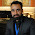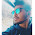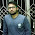## Image Processing : Morphology based Segmentation using MATLAB with program code

Segmentation or contouring could be also obtained using morphological operations. Segmentation subdivides an image into its constituent regions or objects. The level to which the subdivision is carried depends on the problem being solved. That is, segmentation should stop when the objects of interest in an application have been isolated. For example, in the automated inspection of electronic assemblies, interest lies in analyzing images of the products with the objective of determining the presence or absence of specific anomalies, such as missing components or broken connection paths. There is no point in carrying segmentation past the level of detail required to identify those elements.

Structuring Element :

The structuring element consists of a pattern specified as the coordinates of a number of discrete points relative to some origin.
The origin is marked by a ring around that point.
Erosion :

Erosion of A by B is defined as:
Where A is the image and B is the structural element.

One simple application is eliminating irrelevant detail from a binary image.Structuring elementErosion
Dilation :

Dilation of A by B is defined as:

Where B is the structural element and A is the image.

Dilation has the effect of increasing the size of an object.Dilation
Opening :

The opening of set A by the structuring element B is defined by:

Opening generally smoothes the contour of an object, breaks narrow isthmuses, and eliminates thin protrusions.

Very simply, an opening is defined as an erosion followed by a dilation using the same structuring element for both operations.

Note *: opening cannot be done multiple times

Closing :

The closing of set A by the structuring element B is defined by:

Closing also tends to smooth sections of contours but, as opposed to opening, it generally fuses narrow breaks and long thin gulfs, eliminates small holes, and fills gaps in the contour.

It is defined simply as a dilation followed by an erosion using the same structuring element for both operations.

Note *: closing cannot be done multiple timesClosing

Thinning and Thickening :

Thinning is a morphological operation that is used to remove selected foreground pixels from binary images, somewhat like erosion or opening

The thinning operation is related to the hit-and-miss transform.where the subtraction is a logical subtraction defined by :The choice of structuring element determines under what situations a foreground pixel will be set to background, and hence it determines the application for the thinning operation.

Example of skeletonization  through thinningoriginal                                  skeletonized

Thickening

Thickening is a morphological operation that is used to grow selected regions of foreground pixels in binary images, somewhat like dilation or closing:Thus the thickened image consists of the original image plus any additional foreground pixels switched on by the hit-and-miss transform.

Example

Structuring elements for determining the convex hull using thickening. During each iteration of the thickening, each element should be used in turn, and then in each of their 90° rotations, giving 8 effective structuring elements in total.original                thick
MATLAB PROGRAM :
```Thresh=120; % threshold
filename= 'C2L_M.jpg'; % the file to be read
im=imresize(im, [256 256]);
figure(1);
Imagesc(im);  % display
axis('square');
colormap('gray');

im1=uint8(im);
LEVEL=Thresh/255.0;
BW = IM2BW(im1,LEVEL); %converts the intensity image I to black and white.

% dilation

K3=ones(3); K5=ones(5); K7=ones(7); K9=ones(9);

B3=imdilate(BW,K3);
B5=imdilate(BW,K5);
B7=imdilate(BW,K7);
B9=imdilate(BW,K9);

figure(2);
Imagesc(B3);  % display
axis('square');
colormap('gray');

figure(3);
Imagesc(B5);  % display
axis('square');
colormap('gray');

figure(4);
Imagesc(B7);
axis('square');
colormap('gray');

figure(5);
Imagesc(B9);
axis('square');
colormap('gray');

im1=uint8(im);
BW = IM2BW(im1,LEVEL);

% erosion

K3=ones(3); K5=ones(5); K7=ones(7); K9=ones(9);

B3=imerode(BW,K3);
B5=imerode(BW,K5);
B7=imerode(BW,K7);
B9=imerode(BW,K9);

figure(6);
Imagesc(B3);
axis('square');
colormap('gray');

figure(7);
Imagesc(B5);
axis('square');
colormap('gray');

figure(8);
Imagesc(B7);
axis('square');
colormap('gray');

figure(9);
Imagesc(B9);
axis('square');
colormap('gray');

im1=uint8(im);
BW = IM2BW(im1,LEVEL);

figure(10);
Imagesc(BW);
axis('square');
colormap('gray');

% opening

op=bwmorph(BW,'open');
figure(11);
Imagesc(op);
axis('square');
colormap('gray');

im1=uint8(im);
BW = IM2BW(im1,LEVEL); ```
```% closing

op=bwmorph(BW,'close');
figure(12);
Imagesc(op);
axis('square');
colormap('gray');

im1=uint8(im);
BW = IM2BW(im1,LEVEL);

% thinning

op=bwmorph(BW,'thin');
figure(13);
Imagesc(op);
axis('square');
colormap('gray');

im1=uint8(im);
BW = IM2BW(im1,LEVEL);

% thickening

op=bwmorph(BW,'thick');
figure(14);
Imagesc(op);
axis('square');
colormap('gray');
```Image Used in the Program

NOTE :

*To run the code you will need MATLAB.

1.2.3.